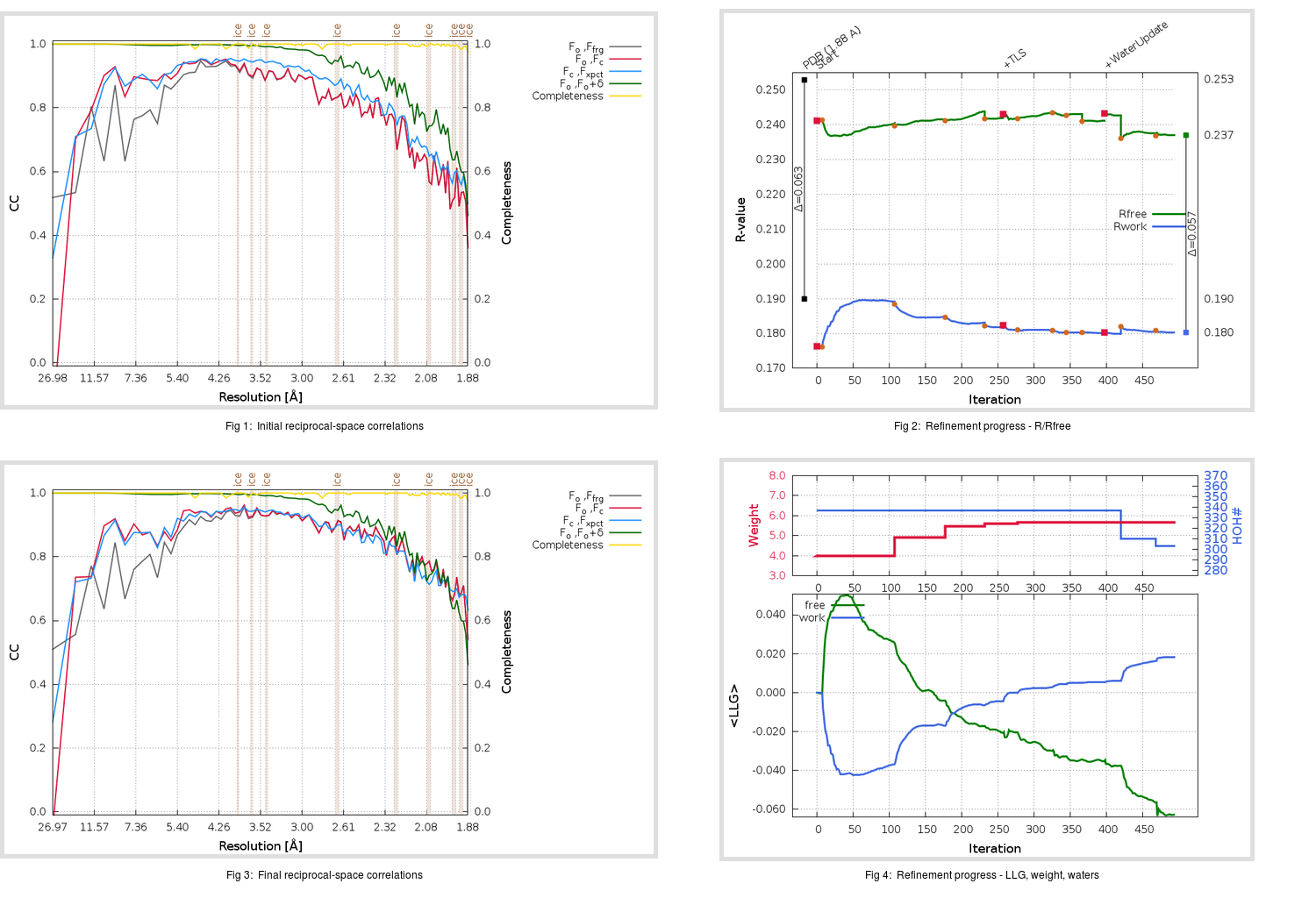Content:

```    Diffraction limits & principal axes of ellipsoid fitted to diffraction cut-off surface:
2.036         0.9177   0.0000  -0.3973       0.970 a* - 0.244 c*
1.787         0.0000   1.0000   0.0000       b*
1.802         0.3973   0.0000   0.9177       0.782 a* + 0.623 c*
```

## Deposited

` `
 Date deposited Date data collection Resolution R, Rfree 20200315 20200304 1.88 0.1870 0.2530

Molprobity (CCP4 7.0 version) summary:

```Ramachandran outliers =   0.33 %
favored =  96.69 %
Rotamer outliers      =   1.14 %
C-beta deviations     =     1
Clashscore            =   5.70
RMS(bonds)            =   0.0141
RMS(angles)           =   1.91
MolProbity score      =   1.56
Resolution            =   1.88
R-work                =   0.1870
R-free                =   0.2530
```

```Number of waters      =   337

<B> (all atoms) =   29.04 ( sd =   10.51 ) for       2735 non-hydrogen atoms
<B>   (protein) =   27.59 ( sd =    9.48 ) for       2370 non-hydrogen atoms
<B>     (water) =   38.48 ( sd =   12.45 ) for        337 non-hydrogen atoms
<B>    (others) =   37.94 ( sd =    9.50 ) for         28 non-hydrogen atoms

B min/max       (all non-hydrogen atoms) =   15.21 /  396.95
B min/max   (protein non-hydrogen atoms) =   15.42 /   83.70
B min/max     (water non-hydrogen atoms) =   15.21 /  396.95
B min/max     (other non-hydrogen atoms) =   28.88 /   55.47
```

## BUSTER (re-)refinement

` `

Molprobity (CCP4 7.0 version) summary:

```Ramachandran outliers =   0.33 %
favored =  98.01 %
Rotamer outliers      =   1.89 %
C-beta deviations     =     0
Clashscore            =   2.96
RMS(bonds)            =   0.0115
RMS(angles)           =   1.60
MolProbity score      =   1.30
Resolution            =   1.88
R-work                =   0.1804
R-free                =   0.2371
```

```Number of waters      =   303

<B> (all atoms) =   29.66 ( sd =    8.45 ) for       2701 non-hydrogen atoms
<B>   (protein) =   28.24 ( sd =    7.08 ) for       2370 non-hydrogen atoms
<B>     (water) =   39.97 ( sd =   10.35 ) for        303 non-hydrogen atoms
<B>    (others) =   37.48 ( sd =   11.18 ) for         28 non-hydrogen atoms

B min/max       (all non-hydrogen atoms) =   15.36 /   67.45
B min/max   (protein non-hydrogen atoms) =   17.78 /   60.73
B min/max     (water non-hydrogen atoms) =   15.36 /   67.45
B min/max     (other non-hydrogen atoms) =   29.71 /   55.21
```

Refinement progression:Results:

` `
 File Remark 5RER_aB_refine.01_03_refine.pdb.gz exact refinement commands are in header 5RER_aB_refine.01_03_refine.mtz.gz including original deposited data and several re-refinement map coefficients 5RER_aB_refine.01_03_BUSTER_model.cif.gz including any non-standard compound restraints 5RER_aB_refine.01_03_BUSTER_refln.cif.gz